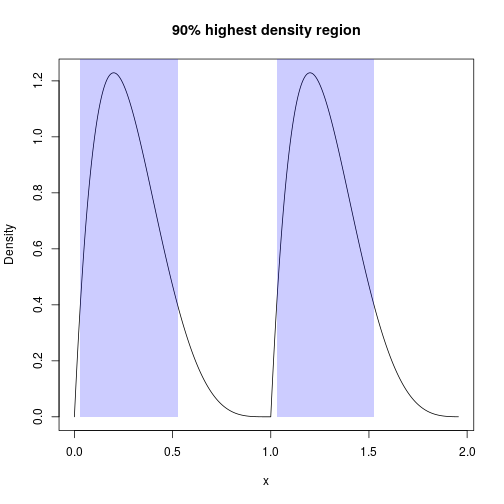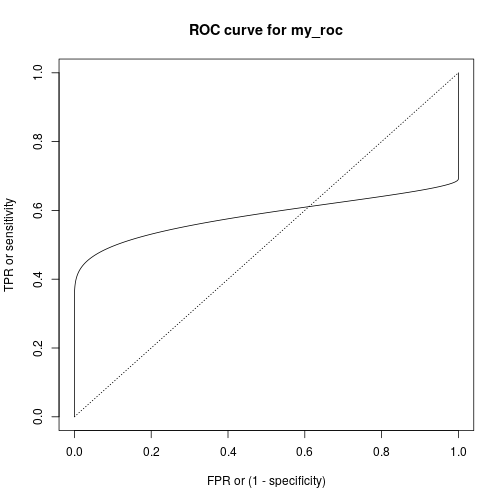Concept of summary functions is to take one or more pdqr-function(s) and return a summary value (which shouldn't necessarily be a number). Argument `method` is used to choose function-specific algorithm of computation.

Note that some summary functions can accumulate pdqr approximation error (like `summ_moment()` for example). For better precision increase number intervals for piecewise-linear density using either `n` argument for `density()` in `new_*()` or `n_grid` argument in `as_*()`.

We will use the following distributions throughout this vignette:

``````my_beta <- as_d(dbeta, shape1 = 2, shape2 = 5)
my_norm <- as_d(dnorm, mean = 0.5)
my_beta_mix <- form_mix(list(my_beta, my_beta + 1))
``````

Although they both are continuous, discrete distributions are also fully supported.

## Basic numerical summary

### Center

``````# Usage of `summ_center()`
summ_center(my_beta, method = "mean")
#>  0.2857136
summ_center(my_beta, method = "median")
#>  0.2644498
summ_center(my_beta, method = "mode")
#>  0.2000014

# Usage of wrappers
summ_mean(my_beta)
#>  0.2857136
summ_median(my_beta)
#>  0.2644498
summ_mode(my_beta)
#>  0.2000014

# `summ_mode()` can compute local modes instead of default global
summ_mode(my_beta_mix, method = "local")
#>  0.2000014 1.2000014
``````

``````# Usage of `summ_spread()`
#>  0.1597178
#>  0.02550977
#>  0.2283162
#>  0.1122417
#>  0.955573

# Usage of wrappers
summ_sd(my_beta)
#>  0.1597178
summ_var(my_beta)
#>  0.02550977
summ_iqr(my_beta)
#>  0.2283162
#>  0.1122417
summ_range(my_beta)
#>  0.955573
``````

### Moments

`summ_moment()` has extra arguments for controlling the nature of moment (which can be combined):

``````summ_moment(my_beta, order = 3)
#>  0.0476182
summ_moment(my_beta, order = 3, central = TRUE)
#>  0.002429287
summ_moment(my_beta, order = 3, standard = TRUE)
#>  11.68727
summ_moment(my_beta, order = 3, absolute = TRUE)
#>  0.0476182
``````

There are wrappers for most common moments: skewness and kurtosis:

``````summ_skewness(my_beta)
#>  0.596237

# This by default computes excess kurtosis
summ_kurtosis(my_beta)
#>  -0.1202127

# Use `excess = FALSE` to compute non-excess kurtotsis
summ_kurtosis(my_beta, excess = FALSE)
#>  2.879787
``````

### Quantiles

`summ_quantile(f, probs)` is essentially a more strict version of `as_q(f)(probs)`:

``````summ_quantile(my_beta, probs = seq(0, 1, by = 0.25))
#>  0.0000000 0.1611628 0.2644498 0.3894790 0.9555730
``````

### Entropy

`summ_entropy()` computes differential entropy (which can be negative) for “continuous” type pdqr-functions, and information entropy for “discrete”:

``````summ_entropy(my_beta)
#>  -0.4845421
summ_entropy(new_d(1:10, type = "discrete"))
#>  2.302585
``````

`summ_entropy2()` computes entropy based summary of relation between a pair of distributions. There are two methods: default “relative” (for relative entropy which is Kullback-Leibler divergence) and “cross” (for cross-entropy). It handles different supports by using `clip` (default `exp(-20)`) value instead of 0 during `log()` computation. Order of input does matter: `summ_entropy2()` uses support of the first pdqr-function as integration/summation reference.

``````summ_entropy2(my_beta, my_norm)
#>  1.439193
summ_entropy2(my_norm, my_beta)
#>  11.61849
summ_entropy2(my_norm, my_beta, clip = exp(-10))
#>  5.289639
summ_entropy2(my_beta, my_norm, method = "cross")
#>  0.9546508
``````

## Regions

Distributions can be summarized with regions: union of closed intervals. Region is represented as data frame with rows representing intervals and two columns “left” and “right” with left and right interval edges respectively.

### Single interval

`summ_interval()` summarizes input pdqr-function with single interval based on the desired coverage level supplied in argument `level`. It has three methods:

• Default “minwidth”: interval with total probability of `level` that has minimum width.
• “percentile”: `0.5*(1-level)` and `1 - 0.5*(1-level)` quantiles.
• “sigma”: interval centered at the mean of distribution. Left and right edges are distant from center by the amount of standard deviation multiplied by `level`'s critical value (computed from normal distribution). Corresponds to classical confidence interval of sample based on assumption of normality.
``````summ_interval(my_beta, level = 0.9, method = "minwidth")
#>         left     right
#> 1 0.03015543 0.5252921
summ_interval(my_beta, level = 0.9, method = "percentile")
#>         left     right
#> 1 0.06284986 0.5818016
summ_interval(my_beta, level = 0.9, method = "sigma")
#>         left    right
#> 1 0.02300124 0.548426
``````

### Highest density region

`summ_hdr()` computes highest density region (HDR) of a distribution: set of intervals with the lowest total width among all sets with total probability not less than an input `level`. With unimodal distribution it is essentially the same as `summ_interval()` with “minwidth” method.

``````# Unimodal distribution
summ_hdr(my_beta, level = 0.9)
#>         left     right
#> 1 0.03013169 0.5253741

# Multimodal distribution
summ_hdr(my_beta_mix, level = 0.9)
#>         left     right
#> 1 0.03015315 0.5252785
#> 2 1.03015315 1.5252785

# Compare this to single interval of minimum width
summ_interval(my_beta_mix, level = 0.9, method = "minwidth")
#>         left    right
#> 1 0.05125316 1.448297
``````

### Work with region

There is a `region_*()` family of functions which helps working with them:

``````beta_mix_hdr <- summ_hdr(my_beta_mix, level = 0.9)
beta_mix_interval <- summ_interval(my_beta_mix, level = 0.9)

# Test if points are inside region
region_is_in(beta_mix_hdr, x = seq(0, 2, by = 0.5))
#>  FALSE  TRUE FALSE  TRUE FALSE

# Compute total probability of a region
region_prob(beta_mix_hdr, f = my_beta_mix)
#>  0.899991

# Pdqr-function doesn't need to be the same as used for computing region
region_prob(beta_mix_hdr, f = my_norm)
#>  0.336239

# Compute height of region: minimum value of d-function inside region
region_height(beta_mix_hdr, f = my_beta_mix)
#>  0.400163

# Compute width of region: sum of interval widths
region_width(beta_mix_hdr)
#>  0.9902507

# Compare widths with single interval
region_width(beta_mix_interval)
#>  1.397043

# Draw region on existing plot
plot(my_beta_mix, main = "90% highest density region")
region_draw(beta_mix_hdr)
``````## Distance

Function `summ_distance()` takes two pdqr-functions and returns a distance between two distributions they represent. Many methods of computation are available. This might be useful for doing comparison statistical inference.

``````# Kolmogorov-Smirnov distance
summ_distance(my_beta, my_norm, method = "KS")
#>  0.419766

# Total variation distance
summ_distance(my_beta, my_norm, method = "totvar")
#>  0.730451

# Probability of one distribution being bigger than other, normalized to [0;1]
summ_distance(my_beta, my_norm, method = "compare")
#>  0.1678761

# Wassertein distance: "average path density point should travel while
# transforming from one into another"
summ_distance(my_beta, my_norm, method = "wass")
#>  0.6952109

# Cramer distance: integral of squared difference of p-functions
summ_distance(my_beta, my_norm, method = "cramer")
#>  0.1719884

# "Align" distance: path length for which one of distribution should be "moved"
# towards the other so that they become "aligned" (probability of one being
# greater than the other is 0.5)
summ_distance(my_beta, my_norm, method = "align")
#>  0.2147014

# "Entropy" distance: `KL(f, g) + KL(g, f)`, where `KL()` is Kullback-Leibler
# divergence. Usually should be used for distributions with same support, but
# works even if they are different (with big numerical penalty).
summ_distance(my_beta, my_norm, method = "entropy")
#>  13.05768
``````

## Separation and classification

### Separation

Function `summ_separation()` computes a threshold that optimally separates distributions represented by pair of input pdqr-functions. In other words, `summ_separation()` solves a binary classification problem with one-dimensional linear classifier: values not more than some threshold are classified as one class, and more than threshold - as another. Order of input functions doesn't matter.

``````summ_separation(my_beta, my_norm, method = "KS")
#>  0.6175981
summ_separation(my_beta, my_norm, method = "F1")
#>  0.007545242
``````

### Classification metrics

Functions `summ_classmetric()` and `summ_classmetric_df()` compute metric(s) of classification setup, similar to one used in `summ_separation()`. Here classifier threshold should be supplied and order of input matters. Classification is assumed to be done as follows: any x value not more than threshold value is classified as “negative”; if more - “positive”. Classification metrics are computed based on two pdqr-functions: `f`, which represents the distribution of values which should be classified as “negative” (“true negative”), and `g` - the same for “positive” (“true positive”).

``````# Many threshold values can be supplied
thres_vec <- seq(0, 1, by = 0.2)
summ_classmetric(f = my_beta, g = my_norm, threshold = thres_vec, method = "F1")
#>  0.5138193 0.5436321 0.6089061 0.6131005 0.5522756 0.4715757

# In `summ_classmetric_df()` many methods can be supplied
summ_classmetric_df(
f = my_beta, g = my_norm, threshold = thres_vec, method = c("GM", "F1", "MCC")
)
#>   threshold        GM        F1         MCC
#> 1       0.0 0.0000000 0.5138193 -0.42709306
#> 2       0.2 0.4614729 0.5436321 -0.03892981
#> 3       0.4 0.6433485 0.6089061  0.31475763
#> 4       0.6 0.6643221 0.6131005  0.48370132
#> 5       0.8 0.6176386 0.5522756  0.48316011
#> 6       1.0 0.5554612 0.4715757  0.42709306
``````

With `summ_roc()` and `summ_rocauc()` one can compute data frame of ROC curve points and ROC AUC value respectively. There is also a `roc_plot()` function for predefined plotting of ROC curve.

``````my_roc <- summ_roc(my_beta, my_norm)
#>   threshold fpr          tpr
#> 1  5.253425   0 0.000000e+00
#> 2  5.243918   0 4.811646e-08
#> 3  5.234412   0 9.845779e-08
#> 4  5.224905   0 1.511167e-07
#> 5  5.215398   0 2.061949e-07
#> 6  5.205891   0 2.637984e-07
summ_rocauc(my_beta, my_norm)
#>  0.583938
roc_plot(my_roc)
``````## Ordering

'pdqr' has functions that can order set of distributions. They are `summ_order()`, `summ_sort()`, and `summ_rank()`, which are analogues of `order()`, `sort()`, and `rank()` respectively. They take a list of pdqr-functions as input, establish their ordering based on specified method, and return the desired output.

There are two sets of methods:

• Method “compare” uses the following ordering relation: pdqr-function `f` is greater than `g` if and only if `P(f >= g) > 0.5`, or in 'pdqr' code `summ_prob_true(f >= g) > 0.5`. This method orders input based on this relation and `order()` function. Notes:
• This relation doesn't strictly define ordering because it is not transitive. It is solved by first preordering input list based on method “mean” and then calling `order()`.
• Because comparing two pdqr-functions can be time consuming, this method becomes rather slow as number of distributions grows. To increase computation speed (sacrificing a little bit of approximation precision), use less intervals in piecewise-linear approximation of density for “continuous” types of pdqr-functions.
• Methods “mean”, “median”, and “mode” are based on `summ_center()`: ordering of distributions is defined as ordering of corresponding measures of distribution's center.
``````# Here the only clear "correct" ordering is that `a <= b`.
f_list <- list(a = my_beta, b = my_beta + 1, c = my_norm)

# Returns an integer vector representing a permutation which rearranges f_list
# in desired order
summ_order(f_list, method = "compare")
#>  1 3 2

# In this particular case of `f_list` all orderings agree with each other, but
# generally this is not the case: for any pair of methods there is a case
# when they disagree with each other
summ_order(f_list, method = "mean")
#>  1 3 2
summ_order(f_list, method = "median")
#>  1 3 2
summ_order(f_list, method = "mode")
#>  1 3 2

# Use `decreasing = TRUE` to sort decreasingly
summ_order(f_list, method = "compare", decreasing = TRUE)
#>  2 3 1

# Sort list
summ_sort(f_list)
#> \$a
#> Density function of continuous type
#> Support: ~[0, 0.95557] (10000 intervals)
#>
#> \$c
#> Density function of continuous type
#> Support: ~[-4.25342, 5.25342] (10000 intervals)
#>
#> \$b
#> Density function of continuous type
#> Support: ~[1, 1.95557] (10000 intervals)
summ_sort(f_list, decreasing = TRUE)
#> \$b
#> Density function of continuous type
#> Support: ~[1, 1.95557] (10000 intervals)
#>
#> \$c
#> Density function of continuous type
#> Support: ~[-4.25342, 5.25342] (10000 intervals)
#>
#> \$a
#> Density function of continuous type
#> Support: ~[0, 0.95557] (10000 intervals)

# Rank elements: 1 indicates "the smallest", `length(f_list)` - "the biggest"
summ_rank(f_list)
#> a b c
#> 1 3 2
``````

## Other

Functions `summ_prob_true()` and `summ_prob_false()` should be used to extract probabilities from boolean pdqr-functions: outputs of comparing basic operators (like `>=`, `==`, etc.):

``````summ_prob_true(my_beta >= my_norm)
#>  0.416062
summ_prob_false(my_beta >= 2*my_norm)
#>  0.6391
``````

`summ_pval()` computes p-value(s) of observed statistic(s) based on the distribution. You can compute left, right, or two-sided p-values with methods “left”, “right”, and “both” respectively. By default multiple input values are adjusted for multiple comparisons (using stats::p.adjust()):

``````# By default two-sided p-value is computed
summ_pval(my_beta, obs = 0.7)
#>  0.02186803
summ_pval(my_beta, obs = 0.7, method = "left")
#>  0.989066
summ_pval(my_beta, obs = 0.7, method = "right")
#>  0.01093401

# Multiple values are adjusted with `p.adjust()` with "holm" method by default
obs_vec <- seq(0, 1, by = 0.1)
summ_pval(my_beta, obs = obs_vec)
#>   0.0000000000 1.0000000000 1.0000000000 1.0000000000 1.0000000000
#>   1.0000000000 0.4915085377 0.1530761780 0.0255840348 0.0009720023
#>  0.0000000000